## 5.2.4 Low-Discrepancy Sampling

In some applications, selecting points that align with the coordinate axis may be undesirable. Therefore, extensive sampling theory has been developed to determine methods that avoid alignments while distributing the points uniformly. In sampling-based motion planning, grids sometimes yield unexpected behavior because a row of points may align nicely with a corridor in. In some cases, a solution is obtained with surprisingly few samples, and in others, too many samples are necessary. These alignment problems, when they exist, generally drive the variance higher in computation times because it is difficult to predict when they will help or hurt. This provides motivation for developing sampling techniques that try to reduce this sensitivity.

Discrepancy theory and its corresponding sampling methods were developed to avoid these problems for numerical integration . Letbe a measure space, such as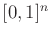. Let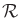be a collection of subsets ofthat is called a range space. In most cases,is chosen as the set of all axis-aligned rectangular subsets; hence, this will be assumed from this point onward. With respect to a particular point set,, and range space,, the discrepancy  forsamples is defined as (see Figure 5.7)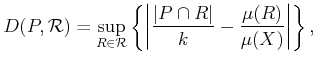(5.22)

in which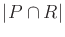denotes the number of points in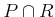. Each term in the supremum considers how wellcan be used to estimate the volume of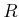. For example, if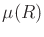is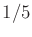, then we would hope that aboutof the points infall into. The discrepancy measures the largest volume estimation error that can be obtained over all sets in.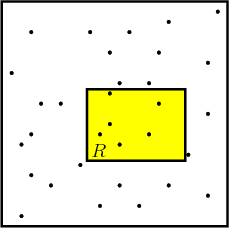Subsections
Steven M LaValle 2012-04-20